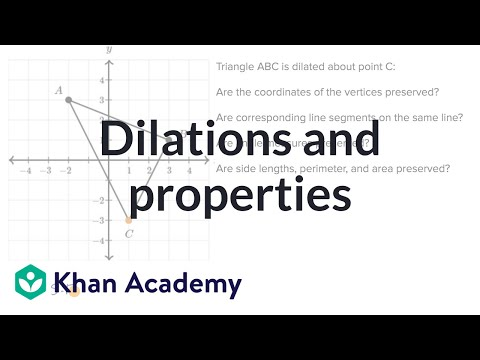Video

# Dilations: scale factor (Full video)

Description: Find the scale factor of a dilation that maps a given figure to another one. We are told that pentagon A'B'C'D'E', which is in red right over here, is the image of pentagon ABCDE under a dilation. So they don't even tell us the center of the dilation, but in order to figure out the scale factor you just have to realize when you do a dilation, the distance between corresponding points will change according to the scale factor. So for example we could look at the distance between point A and point B right over here.

### Other videos you might be interested in### Congruence and similarity: Basic example (Full video)